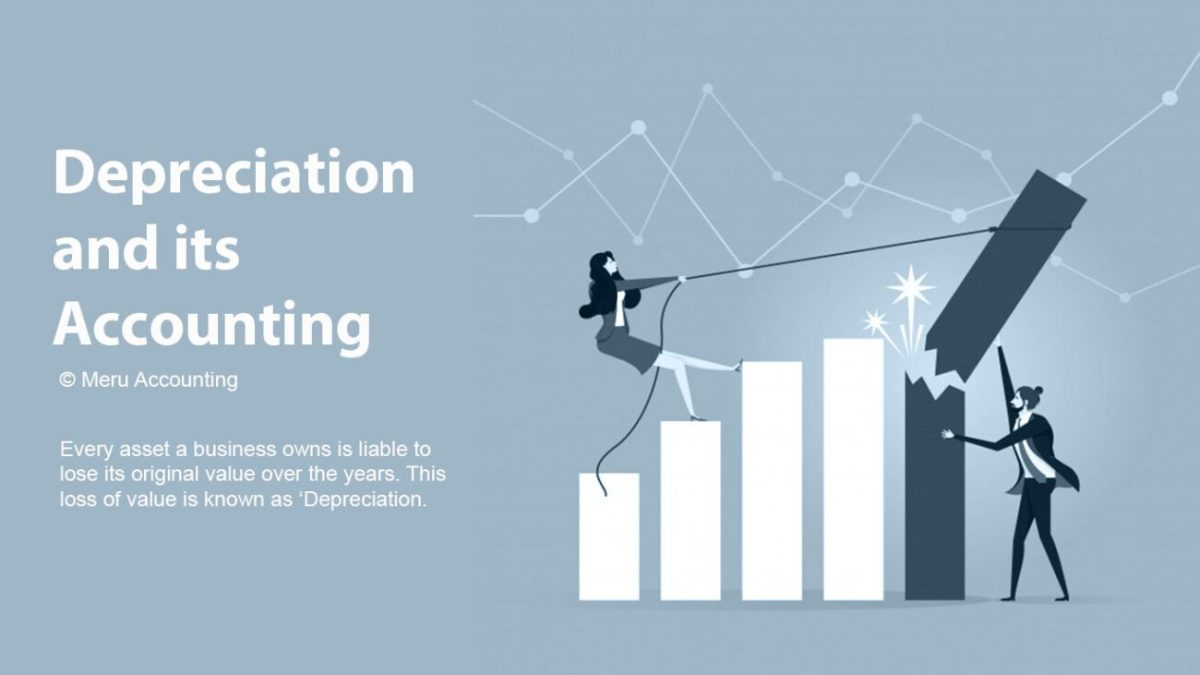Have a question? hello@meruaccounting.com

# What is Depreciation and its Accounting###### Check out how Amanda reduced his Bookkeeping cost?
August 23, 2019## Everything about depreciation

Every asset a business owns is liable to lose its original value over the years. This loss of value is known as ‘Depreciation.

For instance, you purchase any machinery for the production of goods. The business will use that machinery for years and then, eventually be sold off. Until, with each passing year, the cost will decline or can either say will depreciate. If the business chose to sell-off the asset, it must deduct the depreciated value from the cost.

It must be kept in mind that depreciation is only charged on tangible assets like machinery, building, equipment, etc. However, like always, there may be some exceptions like land, whose value increases over the years.

### How To Account For It?

Depreciation is debited every year from the asset account up to the estimated life of the asset. Depreciation is a non-cash transaction, so it does not directly affect the net income of the company. A journal entry is passed every year debiting the depreciated amount from the machinery account. However, the amount that is to be depreciated is calculated when the asset is bought. It is calculated through the following method:

Depreciation = total cost of asset/ estimated life of the asset
Journal entry that is to be passed for depreciation goes like this:
Depreciation a/c Dr.
To Machinery a/c

It is charged up to the years of estimated life until the original value completely gets eliminated.

### Should We Have a Depreciation Fund?

Depreciation fund is the amount equal to the depreciation value that is kept aside from the profit. Although depreciation is a non-cash transaction, it is still a disguised expense for a business. At the end of the depreciation period, a huge expense will arise in the form of a machinery purchase.

It is not mandatory to create any kind of depreciation fund under any accounting rule. However, it is always better to be on the safe side and prevent your business from sudden expenses that will largely affect the revenue.

### Methods Of Calculation Of Depreciation Depreciation on assets can be calculated by four methods:

1. Straight-line method
2. Written down value method
3. Sum of years digit
4. Units of production

Among these, the straight-line method and written down value method are the most prominently used methods in accounting.

1. Straight Line Value Method:
In the straight-line value method, depreciation on asset is charged an equal amount every year up to the estimated life of the asset. This method is also known as a Fixed installment method. After the estimated life is over, the final cost of the asset becomes zero or equal to its scrap value. Depreciation in the straight-line method is only calculated for the time period of the asset actually being in use. If an asset is only used for six months, the depreciation is charged with only that period of time and not for the whole year.

How to calculate depreciation?
Amount of Depreciation = cost of the asset – scrap value/ total estimated life of the asset
Rate of Depreciation = annual depreciation x 100/ cost of the asset

2. Written Down Value Method: Written down value method is also known as the diminishing value method. In this, a fixed percentage is charged every year on the cost of the asset. However, the cost of asset keeps on declining every year. The depreciation in the first year is charged on the original cost at the rate which is pre-decided. But in subsequent years, the depreciation amount is deducted every time the current year’s depreciation is calculated. This method, at starting, may seem to be a bit confusing but is more beneficial as compared to other methods of depreciation.

## Do I Get a Tax Benefit For Depreciation?

Depreciation decreases the value of an asset every year. It does not directly affect the net income or the total cash flow of the business in the current financial period. The depreciation amount will be considered as an expense you are having in the current year. Showing depreciation in the books of accounts will grant you major tax benefits. If you charge a higher rate of depreciation on the asset, it will subsequently decrease your taxable amount due in the period.

### What Is Depreciation Related To Differed Tax?

Differed Tax liability is a situation arises when there is a difference between rate and method of depreciation charged by the company and that fixed by the income tax governing the body of the country. Depreciation is a major example of differed tax liability.